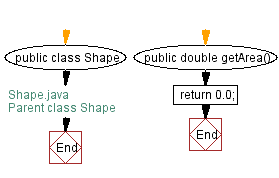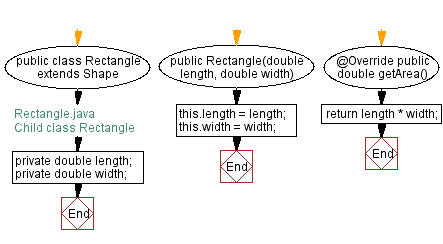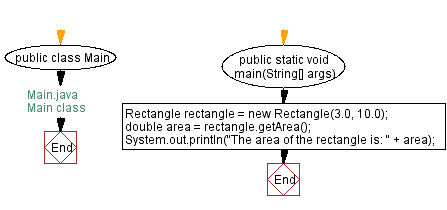﻿ Java Inheritance - Shape class with a method called getArea

# Java Inheritance Programming - Create a class called Shape with a method called getArea and a subclass called Rectangle

## Java Inheritance: Exercise-3 with Solution

Write a Java program to create a class called Shape with a method called getArea(). Create a subclass called Rectangle that overrides the getArea() method to calculate the area of a rectangle.

Sample Solution:

Java Code:

``````// Shape.java
// Parent class Shape
public class Shape {
public double getArea() {
return 0.0;
}
}
```
```
``````// Rectangle.java
// Child class Rectangle
public class Rectangle extends Shape {
private double length;
private double width;

public Rectangle(double length, double width) {
this.length = length;
this.width = width;
}
@Override
public double getArea() {
return length * width;
}
}
``````
``````// Main.java
// Main class
public class Main {
public static void main(String[] args) {
Rectangle rectangle = new Rectangle(3.0, 10.0);
double area = rectangle.getArea();
System.out.println("The area of the rectangle is: " + area);
}
}
``````

Sample Output:

```The area of the rectangle is: 30.0
```

Explanation:

In the above exercise, the Shape class has a single method called getArea() that returns a double value. The Rectangle class is a subclass of Shape and overrides the getArea() method to calculate the area of a rectangle using the formula length x width. The Rectangle class constructor sets length and width values.

Finally in the main() method we create an instance of the Rectangle class and call its getArea() method to get the rectangle's area.

Flowchart:Java Code Editor:

What is the difficulty level of this exercise?

﻿

## Java: Tips of the Day

How do I remove repeated elements from ArrayList?

If you don't want duplicates in a Collection, you should consider why you're using a Collection that allows duplicates. The easiest way to remove repeated elements is to add the contents to a Set (which will not allow duplicates) and then add the Set back to the ArrayList:

```Set<String> set = new HashSet<>(yourList);
yourList.clear();